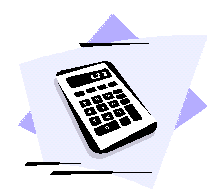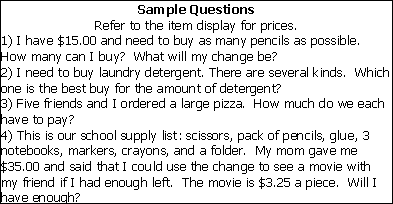##### Math Lesson Plan
Objectives
Students will understand that math is often needed in the real world. They will develop calculator skills while answering a series of questions one might come across in a store.Materials
simple display with items and prices – magazine pictures work well, worksheets with questions that can be answered using the item display, calculators

Procedure
1. Ask the class or small group of students if they have ever bought something at the store? Allow a few students to discuss their experiences briefly.
2. Present the item display, and explain how it is representing a store. Answer any questions the students may have.
3. Present the worksheet of problems and have them work independently, using their calculators to find answers.
4. Discuss the problems when they are done, and have the class or group of students compare their answers as well as the way they found them.
5. Ask them to give some other ways they could have found the answers.
6. This activity could easily be extended by creating a store for the math center the following week. Students could use play money in order to have a hands-on experience at buying products, estimating costs, and making change. They could check their answers with calculators.Closure
Pose this question to the class: If you didn't have calculators, could you still have found the answers? How? Why do you think people use calculators? Are there other times that we might need them besides for buying things?

Evaluation
Were the answers correct? Did the students understand how to use the calculators to solve problems? Were they able to name other uses for calculators in the real world? Do they realize that calculators aren't the only way to solve problems, but just simply an easier way?